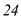# Quiz 15: Managing Projects

PERT and CPM: In order to keep track of the different activities of a project, the following project evaluation tools have to be used. The most predominantly used techniques are PERT and CPM. It is used in project planning and coordination as well. Program evaluation and review technique (PERT) is used to plan, schedule and control different projects such as construction works, design and installation of systems, maintenance work, and research and development processes. It is useful for planning for large and complicated projects. Consider the following data showing immediate predecessor and time estimates for different activities: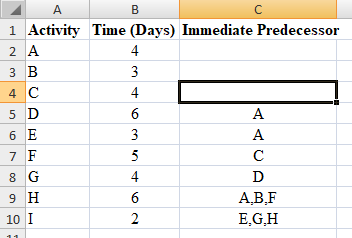a) Network Diagram : Draw a network diagram for the data including each activity as shown below: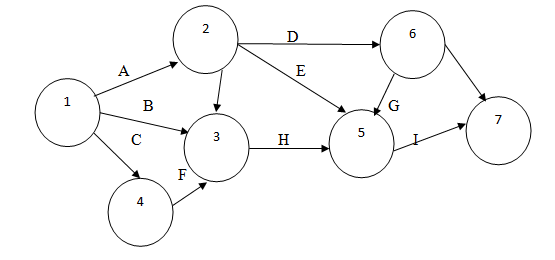b) Scheduling time and Total slack for each activity :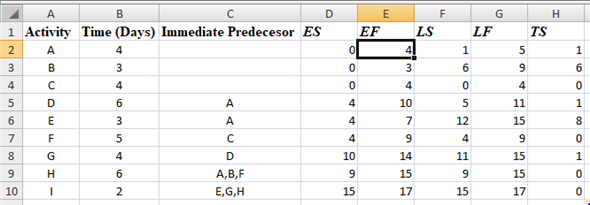The scheduling time for each activity is calculated as follows: Step 1 : List down the different activities in column A, time taken in each activity in column B and the immediate predecessor for each activity in column C. Step 2 : In the column D, write the Earliest Start Time ( ES) of each activity. If an activity has multiple predecessors, then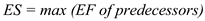.  The Earliest Start time for the subsequent activities will be the Earliest Finish time of the preceding activity. In case there are too many preceding activities, use the finish time of the activity which ends after all the others. Step 3 : In column E, add the time taken in each activity with ES to get the Earliest Finish Time ( EF). The formula used is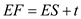Step 4 : In column G, write the Latest Finish Time of each activity ( LF). If an activity has multiple successors,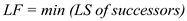. In case of multiple successors, use the smallest late start time from among all of the activities, as the latest finish time. Step 5 : In column F, subtract the time taken in each activity from LF of each activity and obtain LS. The formula used is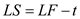Step 6: In column H, subtract ES from LS and obtain the Total Slack value ( TS ). The formula used is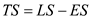c) Critical path and project duration : To identify the critical path of the project, the longest path has to be selected and the path should have no slack. In order to do this, the following diagram is presented. Each circle or node represents an event. An activity occurs between two events. The network diagram is drawn accordingly keeping the predecessors and successors of each activity in mind. Each activity is denoted by an arrow and the number in the brackets is the time taken by each activity. The legend of the diagram is given as follows: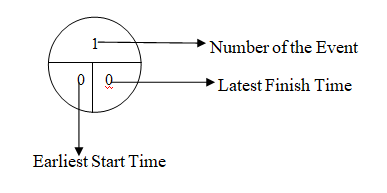Each node is then divided into three parts. The upper half of the node denotes the number of the event and the lower half is sub-divided into two parts. The left hand side of the lower half of the node is the Earliest Start Time ( ES ) and the right hand side is the Latest Finish Time ( LF ). The calculation of ES for all the activities is done from left to right of the network diagram. This is known as Forward Pass. In this, the time taken by each activity is added to the Earliest Start time of each activity. The Earliest Start time from event 1 is considered to be zero. The time taken by activity A is 4 days so the Earliest Start time for event 2 is 4 days (i.e.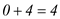). The Earliest Start time from event 4 is 4 days as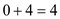. In this manner, the Earliest Start Time is calculated for all the events. In case of multiple predecessors in forward pass, the highest value has to be considered as the Earliest Start time of the event. For example, in event 3, the Earliest Start Time can be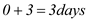(or)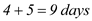. The higher value should be considered i.e. 9 days. On reaching the last event, the calculation of the Latest Finish time of each event has to be done. This is done from right to left. This is known as backward pass. The Earliest Start time of the last event is equal to the Latest Finish time of the last event. The Latest Finish time is then calculated by subtracting the time taken by each activity. For event 5, the Latest Finish time is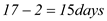. For event 6, the Latest Finish time is either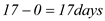(as it is a dummy activity) (or)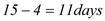. In backward pass, in case of multiple predecessors, the lowest value has to be taken. Therefore, event 6 will take 11 days. In this way, the calculation should be done from event 7 to event 1. The Earliest Start time and the Latest Finish time should be equal to each other at event 1 and event 7 respectively. To identify the critical path, select the activities which have no slack. This can be observed in the activities C, F, H and I. The critical path has been represented in blue arrows in the following diagram.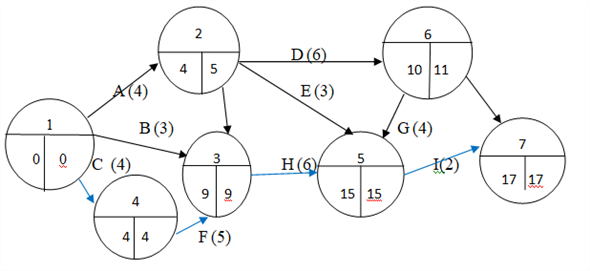The critical path is the longest path in the project that includes the project activities which do not have slack. In the given question, the critical path is CFHI Now, calculate the length of each path by adding the expected time required for completion of each activity present in a path. Project duration is calculated by the sum of the number of days that each individual activity in the critical path will take. In this case,The project duration is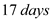. d) Number of workers : If one worker is required for each activity then the resource levelled schedule is prepared as follows: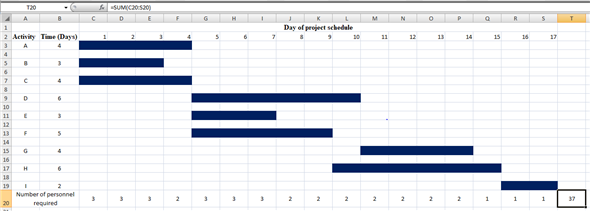Step 1 : List down the different activities and the duration of each activity. Step 2 : Write the number of days that the project takes to be completed. In this case the number of days is 17. Step 3: Fill the number of days taken by each activity under the days of the project schedule. Step 4 : In the row for number of personnel required, enter the sum of the number of people required for all the activities that are carried out in a particular day. Step 5 : Add the total of the number of personnel required per day to get the total number of personnel required for the whole project. The resource levelled schedule shows the number of personnel required on each day of the project. The maximum number of workers required for finishing the project on time is the sum of the number of personnel required on each day of the project. The maximum number of personnel required is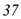PERT and CPM: In order to keep track of the different activities of a project, the following project evaluation tools have to be used. The most predominantly used techniques are PERT and CPM. It is used in project planning and coordination as well. Program evaluation and review technique (PERT) is used to plan, schedule and control different projects such as construction works, design and installation of systems, maintenance work, and research and development processes. It is useful for planning for large and complicated projects. Consider the following data showing immediate predecessor and time estimates for different activities: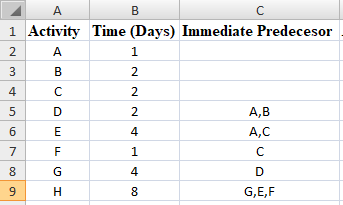a) Network Diagram : Draw a network diagram for the data including each activity as shown below: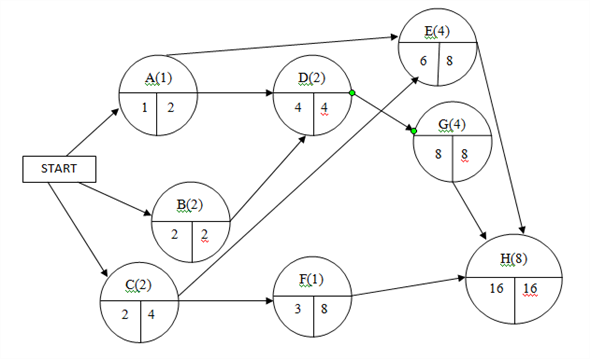b) Scheduling time and Total slack for each activity :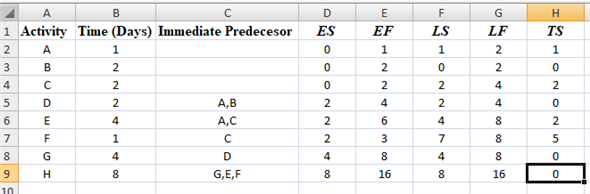The scheduling time for each activity is calculated as follows: Step 1 : List down the different activities in column A, time taken in each activity in column B and the immediate predecessor for each activity in column C. Step 2 : In the column D, write the Earliest Start Time ( ES) of each activity. If an activity has multiple predecessors, then.  The Earliest Start time for the subsequent activities will be the Earliest Finish time of the preceding activity. In case there are too many preceding activities, use the finish time of the activity which ends after all the others. Step 3 : In column E, add the time taken in each activity with ES to get the Earliest Finish Time ( EF). The formula used isStep 4 : In column G, write the Latest Finish Time of each activity ( LF). If an activity has multiple successors,. In case of multiple successors, use the smallest late start time from among all of the activities, as the latest finish time. Step 5 : In column F, subtract the time taken in each activity from LF of each activity and obtain LS. The formula used isStep 6: In column H, subtract ES from LS and obtain the Total Slack value ( TS ). The formula used isc) Critical path and project duration : To identify the critical path of the project, the longest path has to be selected and the path should have no slack. In order to do this, the following diagram is presented. Each circle or node represents an event. An activity occurs between two events. The network diagram is drawn accordingly keeping the predecessors and successors of each activity in mind. Each activity is denoted by an arrow and the number in the brackets is the time taken by each activity. The legend of the diagram is given as follows: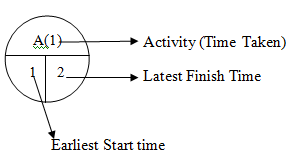Each node is then divided into three parts. The upper half of the node denotes the number of the event and the lower half is sub-divided into two parts. The left hand side of the lower half of the node is the Earliest Start Time ( ES ) and the right hand side is the Latest Finish Time ( LF ). The calculation of ES for all the activities is done from left to right of the network diagram. This is known as Forward Pass. In this, the time taken by each activity is added to the Earliest Start time of each activity. The Earliest Start time from event 1 is considered to be zero. The time taken by activity A is 1 day so the Earliest Start time for event 1 is 1 day (i.e.). In this manner, the Earliest Start Time is calculated for all the events. In case of multiple predecessors in forward pass, the highest value has to be considered as the Earliest Start time of the event. For example, in activity E, the Earliest Start Time can be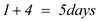(or)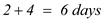. The higher value should be considered i.e. 6 days. On reaching the last event, the calculation of the Latest Finish time of each event has to be done. This is done from right to left. This is known as backward pass. The Earliest Start time of the last event is equal to the Latest Finish time of the last event. The Latest Finish time is then calculated by subtracting the time taken by each activity. For activity G, the Latest Finish time is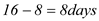. For activity A, the Latest Finish time is either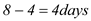(or)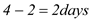. In backward pass, in case of multiple predecessors, the lowest value has to be taken. Therefore, activity A will take 2 days. In this way, the calculation should be done from activity H to start. The Earliest Start time and the Latest Finish time should be equal to each other at the beginning and end of the network diagram, respectively. To identify the critical path, select the activities which have no slack. This can be observed in the activities B, D, G and H The critical path has been represented in blue arrows in the following diagram.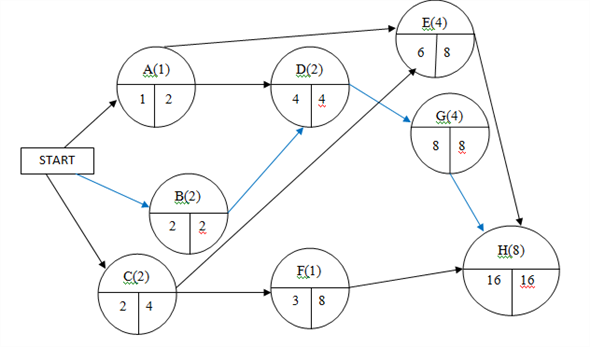The critical path is the longest path in the project that includes the project activities which do not have slack. In the given question, the critical path is BDGH as the activities B, D, G and H do not have slack. Project duration is calculated by the sum of the number of days that each individual activity in the critical path will take. In this case,The project duration is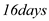d) Number of workers : If one worker is required for each activity then the resource levelled schedule is prepared as follows: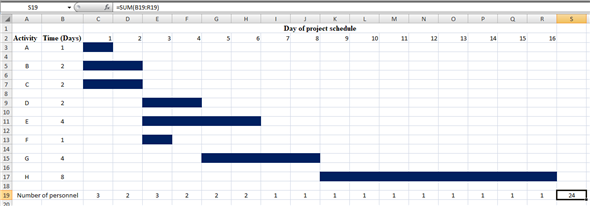Step 1 : List down the different activities and the duration of each activity. Step 2 : Write the number of days that the project takes to be completed. In this case the number of days is 16. Step 3: Fill the number of days taken by each activity under the days of the project schedule. Step 4 : In the row for number of personnel required, enter the sum of the number of people required for all the activities that are carried out in a particular day. Step 5 : Add the total of the number of personnel required per day to get the total number of personnel required for the whole project. The resource levelled schedule shows the number of personnel required on each day of the project. The maximum number of workers required for finishing the project on time is the sum of the number of personnel required on each day of the project. The maximum number of personnel required is